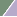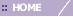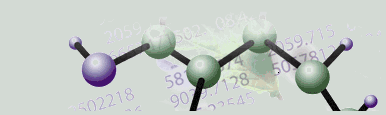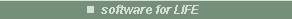# Subtraction of Central Tendency

Overview

Subtraction of central tendency adjusts each sample in a dataset to have a median or mean of zero.

Subtraction of central tendency is typically used to adjust log-ratio values to result in a median (or mean) log-ratio of zero for each sample. This is appropriate, for instance, if the treatment and control dyes in a two-color experiment are incorporated with some bias independent of intensity.

Lowess normalization produces an adjustment almost identical to subtraction of a constant mean if the dye bias is, in fact, independent of intensity. But Lowess is not constrained to produce only a constant correction as subtraction of central tendency is, so it is more general. We therefore recommend Lowess normalization over subtraction of central tendency as a means of normalizing two-color datasets.

Subtraction of Central Tendency Characteristics

• All samples in the dataset are corrected independently.

• This normalization can be applied to complete or incomplete datasets. If either the red or green intensity value is missing for a certain gene, a missing value is placed at the corresponding position in the generated ratio dataset.

Actions

1. Click on a two-color dataset in the Experiments navigator. The item is highlighted.

2. Click on the Normalization toolbar icon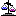, or select Normalize from the Data menu, or right-click the item and select Normalize from the shortcut menu. The first Normalization dialog is displayed.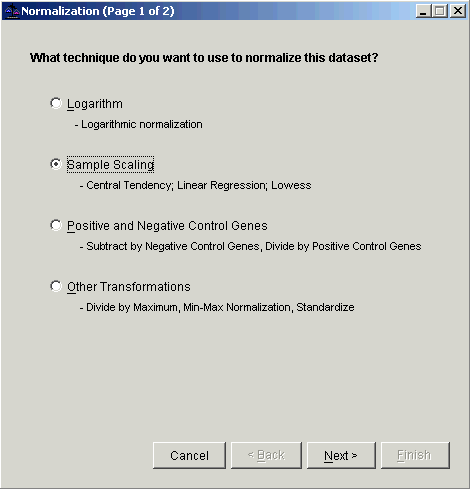3. Select Sample Scaling. The second Normalization dialog is displayed.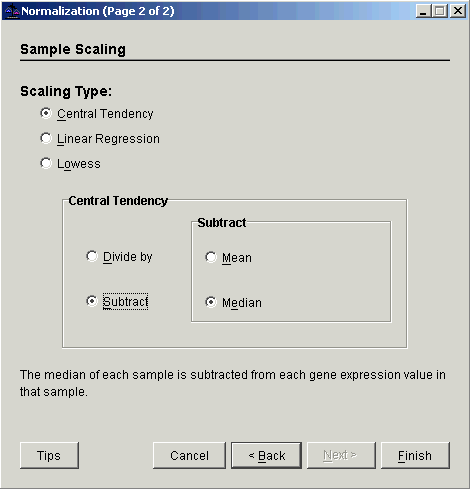4. Select Central Tendency as the Scaling Type.

5. Set the Central Tendency operation to Subtract.

6. Set the Subtract central tendency type to Mean or Median.

7. Click Finish. The Experiment Progress dialog is displayed. It is dynamically updated as the normalization operation is performed.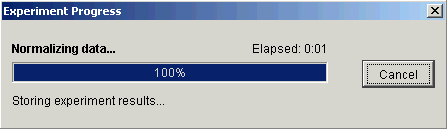• If the operation cannot complete an error message is displayed. The operation will fail, for example, if the mean of any sample is zero or near zero.

• Upon successful completion, a new normalization dataset is added under the original dataset in the Experiments navigator.

Visualization

Once the normalization is complete, a scatter plot can be used to examine each corrected sample.

Related Topics:

Creating an Intensity-Bias Plot of a Sample Ratio

Lowess